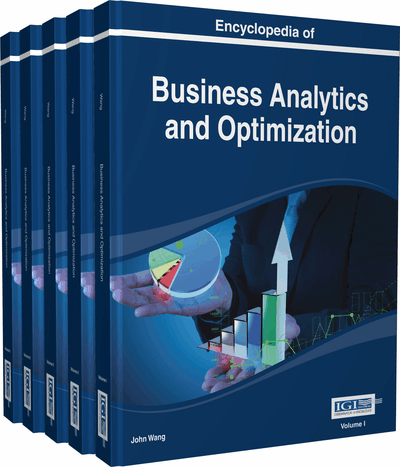# Radial Efficiency Measures in Data Envelopment Analysis

Hirofumi Fukuyama (Fukuoka University, Japan)
DOI: 10.4018/978-1-4666-5202-6.ch177

Top

## Introduction

Data envelopment analysis (DEA) is a non-parametric mathematical programming methodology used to not only evaluate the relative efficiencies of comparable decision making units (DMUs) with multiple inputs and outputs, but also provide efficiency targets for the DMUs. For a visualization of this method, see Figure 1. Since the incept of Charnes, Cooper and Rhodes (1978), DEA has gained increasing popularity in theoretical and applied work. Cooper, Seiford and Tone (2006) provided an in-depth coverage of various DEA models. Cook and Seiford (2009) provided a sketch of the major research thrusts in DEA.

Examples of the DMUs used in real-world DEA applications include manufacturing firms, financial firms, and departments of organizations such as bank branches, hospitals, universities, schools and business firms, as well as regions and countries. Depending on whether inputs or outputs are discretionary or controllable input-oriented or output-oriented radial models can be employed. Both orientations are described in terms of radial efficiency measures as follows:

• Input orientation refers to the emphasis on the evaluated DMU's inputs that can be proportionately expanded without altering the output quantities.

• Output orientation refers to the emphasis on the evaluated DMU’s outputs that can be proportionately reduced without altering the input quantities.

The term “radial efficiency” means that a proportional input reduction or a proportional output augmentation is the main concern in gauging and assessing the efficiency of the DMU. Hence, we can classify DEA models and measures1 into four categories: (1) input-oriented and radial, (2) output-oriented and radial, (3) input-oriented and non-radial, (4) output-oriented and non-radial, and (5) other developments.

In addition to this classification, two scale assumptions are generally utilized: constant returns to scale (CRS) and variable returns to scale (VRS). The CRS assumption implies that a proportional increase in inputs results in a proportional increase in outputs. The VRS assumption implies that the DMUs to be analyzed exhibit increasing, decreasing or constant returns to scale. Although both CRS and VRS assumptions can be implemented in DEA, this chapter considers efficiency measures under the CRS2 assumption, which is adopted by the original work of Charnes, Cooper and Rhodes (1978).

In this chapter, we focus on CRS input-oriented radial efficiency models (measures), in which the observed inputs are minimized given the level of outputs, and hence we deal with the category (1) mentioned above. We refer to the conventional input-oriented radial model (measure) as input-oriented CCR (Charnes-Cooper-Rhodes) or simply CCR model (measure).

The CCR measure evaluates the radial efficiency by searching a point on the weakly efficient frontier rather than the strongly efficient frontier3, and hence the CCR projection point can be on a weakly efficient portion of the frontier. It follows that the CCR measure (the optimal objective function value of the CCR model) does not provide an efficiency score consistent to the strongly efficient frontier due to the use of a non-Archimedean infinitesimal number (not a real number in the standard mathematics), which is smaller than any positive real number (Cooper, Seiford, & Tone 2007, p.88). To find a strongly efficient projection point based on the CCR model, a two-step procedure is often employed. In this procedure, however, an efficiency score is not obtained. In order to make up for this deficiency, we resort to a recently developed measure by Fukuyama and Sekitani (2012a), which has several desirable features as an efficiency measure.

## Key Terms in this Chapter

Linear Programming: A linear program is the optimization of a linear function subject to linear constraints or restrictions.

Normal Cone: The normal cone to the set C at the point is denoted by , and hence is the set of all vectors normal to C at .

Maximal Efficient Face: A face is a maximal efficient face if there is no efficient face such that and .

Data Envelopment Analysis (DEA): DEA is a set of mathematical programming techniques for assessing the relative efficiency of DMUs (decision making units) that transform multiple inputs into multiple outputs.

Face: A face of is a nonempty set of binding hyperplanes of . An efficient face of is contained in , which is the set of all efficient points in .

Mixed-Integer Linear Programming: A mixed integer linear program is the optimization of a linear function subject to linear constraints when some of the variables are integer valued.

Input Requirement Set: The input requirement set is the set of all input bundles required to produce at least a given level of outputs.

## Complete Chapter List

Search this Book:
Reset